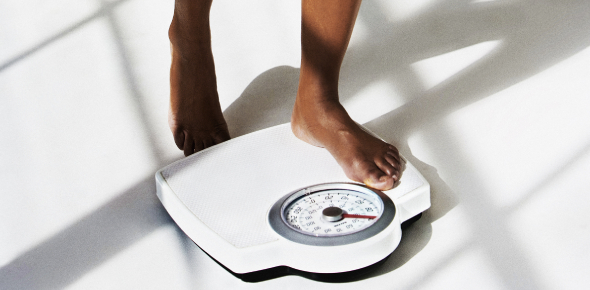# The Weight Quiz: MCQ Trivia Test!

10 Questions | Total Attempts: 249SettingsThe weight quiz: MCQ trivia test! Weight can be measured in grams, kilograms or pounds. It is possible to change one unit of measurement into another, and the quiz below is designed to help see if you can correctly change from one unit to another. Think you can handle it, or do you need more practice? Do take up the quiz and get to find out. All the best!

• 1.
Select the item that is weighed in grams.
• A.

Elephant

• B.

Frog

• C.

T.V.

• D.

Blue whale

• 2.
Select the item that is weighed in kilograms.
• A.

Can of coke

• B.

Book

• C.

Dog

• D.

Candle

• 3.
How many grams in 3 kilograms?
• A.

30 g

• B.

300 g

• C.

3000 g

• D.

30000 g

• 4.
Convert 4300 grams into kilograms.
• A.

43 kg

• B.

4.3 kg

• C.

0.43 kg

• D.

430 kg

• 5.
What is the total of 4 kg + 250 g?
• A.

0.425 kg

• B.

425 kg

• C.

42.5 kg

• D.

4.25 kg

• 6.
What mass is used to weigh a computer?
• A.

Grams

• B.

Kilograms

• C.

Tonnes

• 7.
500g is heavier than 500kg.
• A.

True

• B.

False

• 8.
• A.

True

• B.

False

• 9.
• A.

True

• B.

False

• 10.
If one strawberry weighs 25g, how much would 4 strawberries weigh?
• A.

1kg

• B.

100kg

• C.

100g

Related TopicsBack to top